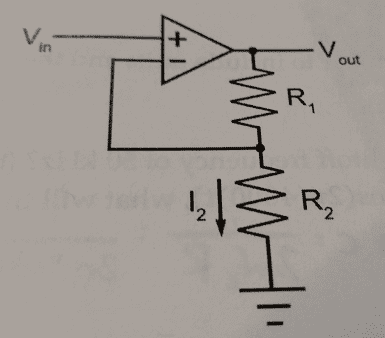Op-amp resister circuit analysis

• Engineering

Homework Statement

Given the ideal op-amp, determine the resistor R2 and the current I2 where Vin=500mV, Vout = 2.5 V, and R1=5kV.Homework Equations

No current passes through op-amps, the op-amp tries to make V+ and V- equal.
Ohm's law, voltage divider equation

The Attempt at a Solution

Since V+ is 500mV, I assumed that the node between R1 and R2 must also be 500mV, as it is connected to V-. So 500mV = 2.5V(R2/(5000Ω+R2)). I got R2 to be 1,250Ω.
Then I found I2=Vout/Req = 2.5V/(1250Ω + 5000Ω) = .4mA.
However, I am unsure because I am not sure if I did the part with the op-amp right; namely, the node between the resistors being set to 500mV.